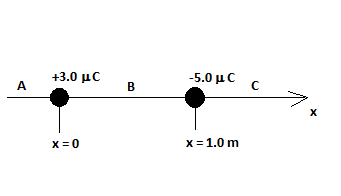# Two point charges are arranged along the x axis as shown. a. At region A, B and C where is the...

## Question:

Two point charges are arranged along the x axis as shown.

a. At region A, B and C where is the electric field equal to zero? Show your analysis.

b. At region A, B and C where is the electric potential equal to zero? Note: At infinity the potential is zero.

c. Calculate the electric field (magnitude and direction) and the electric potential at point P (x = +1.5 m).## Electric Field and Electric Potential:

The electric field created by a positive charge charge points away from the charge but the electric field due to a negative charge points towards the charge. Therefore, electric field due to a negative and a positive charge is zero at a point where the directions of their individual fields are opposite. The net electric potential due to a negative charge and a positive charge can be zero since its an algebraic sum of their individual potentials.

Become a Study.com member to unlock this answer! Create your account

a: Let {eq}E_1 \ and \ E_2 {/eq} be the magnitudes of the electric field due to the positive and the negative charge, respectively. The directions...Calculating Electric Forces, Fields & Potential

from

Chapter 7 / Lesson 6
7.4K

From electric charges come electric forces and electric fields. In this lesson, we will explore how to calculate electric forces between charges, electric fields generated by them, and electric potentials in specific locations because of them.